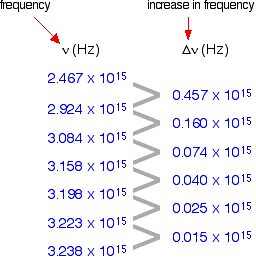# How would you determine the ionization energy of a hydrogen atom (in kJ/mol) if the electron is in its ground state?

Jun 1, 2016

I will suggest two ways you could do this.

#### Explanation:

$\textcolor{b l u e}{\left(1\right)}$

Use the Rydberg expression:

The wavelength $\lambda$ of the emission line in the hydrogen spectrum is given by:

$\frac{1}{\lambda} = R \left[\frac{1}{n} _ {1}^{2} - \frac{1}{n} _ {2}^{2}\right]$

$R$ is the Rydberg Constant and has the value $1.097 \times {10}^{7} {\text{m}}^{- 1}$

${n}_{1}$ is the principle quantum number of the lower energy level

${n}_{2}$ is the principle quantum number of the higher energy level.

The energy levels in hydrogen converge and coalesce:Since the electron is in the ${n}_{1} = 1$ ground state we need to consider series 1. These transitions occur in the u.v part of the spectrum and is known as The Lyman Series.

You can see that as the value of ${n}_{2}$ increases then the value of $\frac{1}{n} _ {2}^{2}$ decreases. At higher and higher values the expression tends to zero until at $n = \infty$ we can consider the electron to have left the atom resulting in an ion.

The Rydberg expression now becomes:

$\frac{1}{\lambda} = R \left[\frac{1}{n} _ {1}^{2} - 0\right] = \frac{R}{n} _ {1}^{2}$

Since ${n}_{1} = 1$ this becomes:

$\frac{1}{\lambda} = R$

$\therefore \frac{1}{\lambda} = 1.097 \times {10}^{7}$

$\therefore \lambda = 9.116 \times {10}^{- 8} \text{m}$

We can now find the frequency and hence the corresponding energy:

$c = \nu \lambda$

$\therefore \nu = \frac{c}{\lambda} = \frac{3 \times {10}^{8}}{9.116 \times {10}^{-} 8} = 3.291 \times {10}^{15} {\text{s}}^{- 1}$

Now we can use the Planck expression:

$E = h \nu$

$\therefore E = 6.626 \times {10}^{- 34} \times 3.291 \times {10}^{15} = 2.18 \times {10}^{- 18} \text{J}$

This is the energy needed to remove 1 electron from 1 hydrogen atom. To find the energy required to ionise 1 mole of H atoms we multiply by the Avogadro Constant:

$E = 2.18 \times {10}^{- 18} \times 6.02 \times {10}^{23} = 13.123 \times {10}^{5} \text{J""/""mol}$

$\textcolor{red}{E = 1312 \text{ ""kJ/mol}}$

$\textcolor{b l u e}{\left(2\right)}$

Use the convergence limit of the u.v spectrum in an experimental method.

You can see from the diagram that the energy levels converge and coalesce to a continuum. This means that the emission lines will converge also.

The frequency at which this happens can give us the ionisation energy.The 1st column shows the frequency of the line.

The second column gives the difference in frequency which is getting less and less.

If you plot $\nu$ against $\Delta \nu$ you get a graph like this:You can actually get 2 lines using upper and lower values. The important point is that they converge to the same point where $\Delta \nu$ tends to zero.

You can the read the convergence limit off the x axis.

This gives $\nu = 3.28 \times {10}^{15} {\text{s}}^{- 1}$ which is very close to the value from method $\textcolor{b l u e}{\left(1\right)}$.

As in method $\textcolor{b l u e}{\left(1\right)}$ we can convert to Joules using the Planck expression:

$E = h \nu = 6.626 \times {10}^{- 34} \times 3.28 \times {10}^{15} = 2.173 \times {10}^{- 18} \text{ ""J}$

So for 1 mole:

$E = 2.173 \times {10}^{- 18} \times 6.02 \times {10}^{23} = 13.08 \times {10}^{5} \text{J/mol}$

$\textcolor{red}{E = 1308 \text{ ""kJ/mol}}$